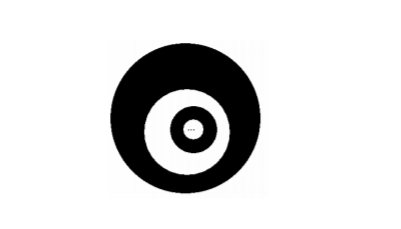r/4 的黑圆，此黑圆里又有一个半径为 r/8 的白圆，如此重复下去，问黑色部分的面积为多少？## 希望前辈们可以在闲暇时间帮忙用递归算法解决一下。非常谢谢！ 晚辈为初学者，望前辈们多多指教。非常谢谢！

### 结尾

public double getArea(double r){
if(r==0){
return 0;
}
return Math.PI*(r*r-(r/2)*(r/2))+getArea(r/4);
}


1

while（r>0）

sum=(-1)^n*3.14r^2【这里的具体实现没学过】
sum=sum+
r=r/2